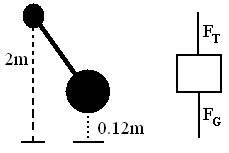# Pendulum and Centripetal Motion Question

• MaZnFLiP

#### MaZnFLiP

[SOLVED] Pendulum and Centripetal Motion Question

Picture and FBDThe Problem/Question
Calculate the speed of a 2.0m length pendulum at the very bottom of the swing if you raise it a vertical height of 0.12m

Relevant equations

$$F_net = F_T + F_G = F_C = m(\frac{V^2}{r})$$

The attempt at a solution

Well, after looking over this problem, I think I'm doing something amazingly wrong.

Looking at My equations, I went from

$$F_C = F_T + mg$$

to

$$m(\frac{V^2}{r}) = F_T + mg$$

From there I got:

$$\frac {V^2}{r} = F_T + g$$ because the masses cancel.

Next:

$$V^2 = gr + F_T$$

After finding $$(-9.81\frac{m}{s}^2)(2.0m) = 19.62$$, I found the square root which was $$4.429 \frac {m}{s}^2$$

The problem is that after looking at the answer sheet, The answer is supposed to be:

$$1.5\frac{m}{s}^2$$

Hi MaZnFLip,

Picture and FBDThe Problem/Question
Calculate the speed of a 2.0m length pendulum at the very bottom of the swing if you raise it a vertical height of 0.12m

Relevant equations

$$F_net = F_T + F_G = F_C = m(\frac{V^2}{r})$$

The attempt at a solution

Well, after looking over this problem, I think I'm doing something amazingly wrong.

Looking at My equations, I went from

$$F_C = F_T + mg$$

At the bottom of the swing, the equation would be $F_c=F_T - mg$, but everywhere else along the swing the tension is not in the opposite direction as the weight, and you would need some trig functions to take that effect into account. Also, you would need to take into account the tangential acceleration that is actually causing the mass to speed up.

Rather than that, I would suggest using conservation of energy for this problem. What does that give?

Umm. The way I learned to use conservation of energy is converting PE since its higher up and when it goes all the way down it turns into KE so PE = KE. That means that MGH = 0.5mv^2 and since the masses cancel that would leave me with GH = 0.5v^2. Multiplying GH together would give me 1.1772. After dividing that with 0.5, I then get 2.3544. Finally, After getting the square root of that, I ended up getting 1.5m/s! Yes! Thank you so much!